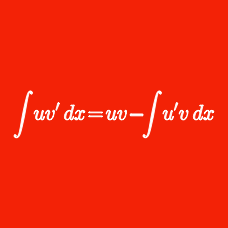Calculus

# Integration Techniques: Level 5 Challenges

$\int_0^1 \left(1-x^2\right)^9 x^9 \, dx$

Let $I$ denote the value of the integral above. What is the sum of digits of $I^{-1}?$

$\left\lfloor \frac{\displaystyle{ \int_{0}^{\infty} e^{-x^2}\, dx}}{\displaystyle{\int_{0}^{\infty} e^{-x^2} \cos 2x \, dx}}\right\rfloor= \ ?$

Define a sequence of functions $\{f_n(x)\}$ as follows: $f_1(x) = 1, \hspace{.4cm} f_{n+1}(x) = \int_0^{\infty}\frac{e^{-tx}}{f_n(t)}dt.$ Evaluate: $\frac{f_{20}(1)f_{21}(1)}{f_{18}(1)f_{19}(1)}$

Suppose a light source is positioned in $\textbf{R}^{2}$ at the origin $(0,0).$ Smooth mirrors are positioned along the lines $y = 0$ and $y = 2$ from $x = 0$ to $x = 10.$ The light source is then oriented so that the beam of light emanating from it makes an angle, chosen uniformly and at random, of between $15^{\circ}$ and $75^{\circ}$ with the positive $x$-axis. The beam is then allowed to reflect back and forth between the two mirrors until it "exits the tunnel", that is, it crosses the line $x = 10.$

If the expected distance that the beam of light travels from its source until it exits the tunnel can be expressed as $\dfrac{10}{\pi}\ln(a + b\sqrt{c}),$ where $a,b,c$ are positive integers with $c$ square-free, then find $a + b + c.$

A function $f(x)$ satisfies the functional equation $f(\tan \theta)=\frac{\sin^2 2\theta}{4}$ for all real $\theta$. If $\displaystyle \int_{0}^{\infty}f(x) \ dx$ is equal to $\dfrac{\pi^a}{b}$ for positive integers $a$ and $b$, then what is the value of $a+b$?

×